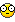## Recommended Posts

Hi,

by extracting data from files or from web-sites it may be, that some entries are double (i.e. user name).

With this function i sort an array and delete doubles in it. With string i can choose case sensitivity.

It works with 2D Array only with 2 occurences in the second dimension. (If you need more -you can expand this script)

Here with an example:

CODE
```#include <array.au3>
Dim \$ar
Dim \$ar2D

\$ar = "Otto"
\$ar = "otto"
\$ar = "Bernd"
\$ar = "Kurt"
\$ar = "otto"
\$ar = "Kurt"
\$ar = "Bernd"
\$ar = "Otto"
\$ar = "Kurt"
\$ar = "bernd"

\$ar2 = \$ar

\$ar2D = "Otto"
\$ar2D = "Otto"
\$ar2D = "Bernd"
\$ar2D = "Kurt"
\$ar2D = "otto"
\$ar2D = "Kurt"
\$ar2D = "Bernd"
\$ar2D = "Otto"
\$ar2D = "Kurt"
\$ar2D = "bernd"

\$ar2D = "meier"
\$ar2D = "meier"
\$ar2D = "McBee"
\$ar2D = "Becker"
\$ar2D = "Meier"
\$ar2D = "Schramm"
\$ar2D = "McBee"
\$ar2D = "Lorenz"
\$ar2D = "meier"
\$ar2D = "Gantzer"

\$ar2D2 = \$ar2D

_ArrayDisplay(\$ar, "unsorted 1D")
_ArraySortDblDel(\$ar)
_ArrayDisplay(\$ar, "w/o case sens 1D")
_ArraySortDblDel(\$ar2,1)
_ArrayDisplay(\$ar2, "case sens 1D")
_ArrayDisplay2D(\$ar2D, "unsorted 2D")
_ArraySortDblDel(\$ar2D,0,0,2,1)
_ArrayDisplay2D(\$ar2D, "w/o case sens 2D")
_ArraySortDblDel(\$ar2D2,1,0,2,1)
_ArrayDisplay2D(\$ar2D2, "case sens 2D")

;----------------------------------------------------------------------------------------------------------------------
; Function      _ArraySortDblDel(ByRef \$ARRAY [, \$CASESENS=0 [, \$iDESCENDING=0 [, \$iDIM=0[ , \$iSORT=0]]]])
;
; Description   - Sorts an 1D/2D Array and delete double entries (2D -> combination by '[n]' and '[n]').
;               - By using string, you can choose case sensitivity.
;               - BaseIndex is 0; sorts the whole array.
;
; Parameter     \$ARRAY:         Array to sort
;   optional    \$CASESENS:      Case sensitivity off or on (default 0)
;   optional    \$iDESCENDING:   Sort ascending/descending (default 0)
;   optional    \$iDIM:          Occurences in the second dimension, eg \$A=0 or \$A=2 (default 0)
;   optional    \$iSORT:         SortIndex by using 2 dimensions (default 0)
;
; Return        ByRef sorted Array without doubles
;
; Requirements  Only 2 occurences in the second dimension
;               #include <array.au3>
;
; Author        BugFix (bugfix@autoit.de)
;----------------------------------------------------------------------------------------------------------------------

Func _ArraySortDblDel(ByRef \$ARRAY, \$CASESENS=0, \$iDESCENDING=0, \$iDIM=0, \$iSORT=0)
Local \$arTmp1D, \$arTmp2D, \$dbl = 0
\$arTmp1D = ""
\$arTmp2D = ""
If \$iDIM = 0 Then \$iDIM = 1
_ArraySort(\$ARRAY,\$iDESCENDING,0,0,\$iDIM,\$iSORT)
Switch \$iDIM
Case 1 ; 1D
For \$i = 0 To UBound(\$ARRAY)-1
\$dbl = 0
For \$k = 0 To UBound(\$arTmp1D)-1
Switch \$CASESENS
Case 0
If \$arTmp1D[\$k] = \$ARRAY[\$i] Then \$dbl = 1
Case 1
If \$arTmp1D[\$k] == \$ARRAY[\$i] Then \$dbl = 1
EndSwitch
Next
If \$dbl = 0 Then
If \$arTmp1D = "" Then
\$arTmp1D = \$ARRAY[\$i]
Else
EndIf
Else
\$dbl = 0
EndIf
Next
\$ARRAY = \$arTmp1D
Case 2 ; 2D
For \$i = 0 To UBound(\$ARRAY)-1
\$dbl = 0
For \$k = 0 To UBound(\$arTmp2D)-1
Switch \$CASESENS
Case 0
If  ( \$arTmp2D[\$k] = \$ARRAY[\$i] ) And _
( \$arTmp2D[\$k] = \$ARRAY[\$i] ) Then \$dbl = 1
Case 1
If  ( \$arTmp2D[\$k] == \$ARRAY[\$i] ) And _
( \$arTmp2D[\$k] == \$ARRAY[\$i] ) Then \$dbl = 1
EndSwitch
Next
If \$dbl = 0 Then
If \$arTmp2D = "" Then
\$arTmp2D = \$ARRAY[\$i]
\$arTmp2D = \$ARRAY[\$i]
Else
ReDim \$arTmp2D[UBound(\$arTmp2D)+1]
\$arTmp2D[UBound(\$arTmp2D)-1] = \$ARRAY[\$i]
\$arTmp2D[UBound(\$arTmp2D)-1] = \$ARRAY[\$i]
EndIf
Else
\$dbl = 0
EndIf
Next
\$ARRAY = \$arTmp2D
EndSwitch
EndFunc ; ==>_ArraySortDblDel

Func _ArrayDisplay2D(\$Array, \$Title="")
Local \$str = ""
For \$i = 0 To UBound(\$Array)-1
If \$i = 0 Then
\$str &= "[" & \$i & "]   = " & \$Array[\$i] & " | " & \$Array[\$i]
Else
\$str &= @LF & "[" & \$i & "]   = " & \$Array[\$i] & " | " & \$Array[\$i]
EndIf
Next
MsgBox(0, \$Title, \$str)
EndFunc```

_ArraySortDblDel.au3

Edited by BugFix

Best Regards BugFix## Create an account

Register a new account

• ### Recently Browsing   0 members

×

• Wiki

• Back

• #### Beta

• Git
• FAQ
• Our Picks
×
• Create New...